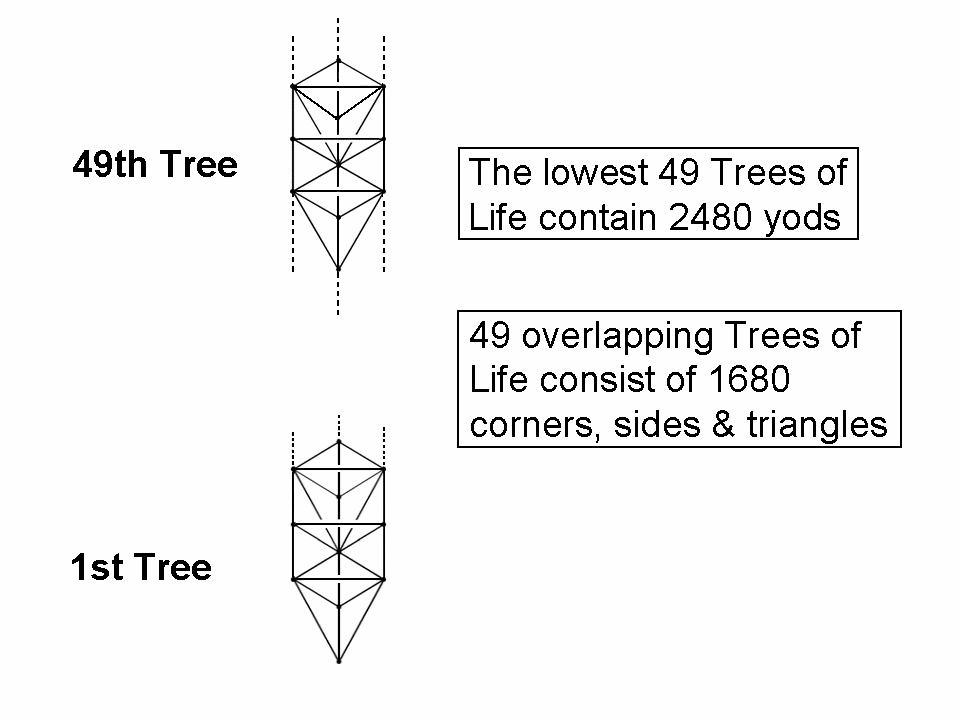<< Previous    1...   14  15    17  18  ...50    Next >>The number Y(n) of yods in the n-tree (the n lowest Trees of Life) is given by

Y(n) = 50n + 30.*

Fittingly, the number value 50 of ELOHIM, the Godname of Binah, the Sephirah heading the Pillar of Judgement and embodying the abstract notion of relationship and organized structure, is the number of yods needed to construct successive Trees of Life:

Y(n+1) − Y(n) = 50.

The lowest 49 Trees have Y(49) = 2480 yods. This is the number of yods in 248 tetractyses. As was discussed in Maps of reality/Cosmic Tree of Life, the lowest 49 Trees map the cosmic physical plane, the lowest of the seven cosmic planes of consciousness. The holistic parameter 248 is embodied therefore in the section of CTOL that corresponds to Malkuth, the lowest Sephirah of Construction. This is a remarkable conjunction of metaphysical ideas and hard, mathematical facts, i.e., the dimension of the largest exceptional Lie group E8 is 248. As a 10-dimensional vector gauge field can be associated with each gauge charge that corresponds to a root of E8, the number 2480 is the number of components of the 248 gauge fields transmitting the superstring force. Appropriately, it is embodied in the Tree of Life mapping of the 49 subplanes of the cosmic physical plane.

The number G(n) of corners, sides & triangles in n overlapping Trees is given by

G(n) = 34n + 14.**

Hence, G(49) = 1680. This is the number of circular turns (circularly polarised oscillations) in each helical whorl of the UPA described paranormally by Besant & Leadbeater and identified by the author in his books and website articles as the subquark state of an E8×E8 heterotic superstring. The lowest 49 Trees embody the dynamical parameter of the gauge symmetry group E8 determining superstring forces, whilst 49 overlapping Trees embody the superstring structural parameter 1680. This is an amazing example of how numbers characterizing the basic physics of the material universe are embodied in sections of CTOL that bear a correspondence to the Sephirah Malkuth signifying this level of reality, namely, the cosmic physical plane mapped by 49 Trees of Life. It is a mathematical fact that conforms to the Hermetic principle: "As above, so below" expounded in the Emerald Tablet of Hermes Trismegistus. It is why the number value 168 of Cholem Yesodoth, the Mundane Chakra of Malkuth, determines this structural parameter, for 1680 is the number of circular turns in a half-revolution of all 10 whorls of the UPA, whilst 168 is the number of turns in a half-revolution of each whorl. Here is remarkable evidence, irrefutable because it is entirely mathematical, that a number with paranormal provenance, namely 1680, appears in the same context as the number 2480, which has a very clear physical meaning in terms of superstring theory. It is convincing evidence that the UPA is, indeed, a state of the superstring and that the number 1680 measures its vibrational form (or, rather, its whorls). It defies all sense of the plausible to dismiss these connections as the result of chance. Sceptics therefore have no way to explain their existence that sounds reasonable and acceptable to unbiased minds. It is not cherry-picking to point that 49 Trees of Life embody the very structural parameter that was paranormally determined over a century ago for particles identified as superstrings by the author over 90 years later in his book ESP of Quarks and Superstrings, whilst the lowest 49 Trees embody the number of space-time components of the 248 gauge fields transmitting superstring forces. It would be cherry picking if both parameters had arisen from different numbers of Trees of Life. But this is not the case here. Once the number of Trees is specified, both numbers appear automatically. Moreover, this particular number of Trees is not arbitrary, because the section of CTOL embodying these numbers is that of the cosmic physical plane, which embodies two numbers of significance to the fundamental units of physical matter, namely E8×E8 heterotic superstrings. The appropriateness of this context is undeniable and cannot be dismissed as yet another coincidence except by those who are ideologically driven enough to be willing to believe in miracles of chance rather than admit that the conjunction of these two numbers is evidence of the genuine nature of Besant's & Leadbeater's micro-psi faculty.

* Proof: the n-tree contains (12n+7) triangles with (6n+5) corners and (16n+9) sides. When the triangles are tetractyses, two hexagonal yods line each side and a hexagonal yod is at the centre of each tetractys. The number of yods in the n-tree = 6n + 5 + 2×(16n+9) + 12n + 7 = 50n + 30.

** Proof: n overlapping Trees of Life consist of (12n+4) triangles with (6n+4) corners and (16n+6) sides. Therefore, they contain (34n+14) corners, sides & triangles.

 << Previous    1...   14  15    17  18  ...50    Next >>

Home